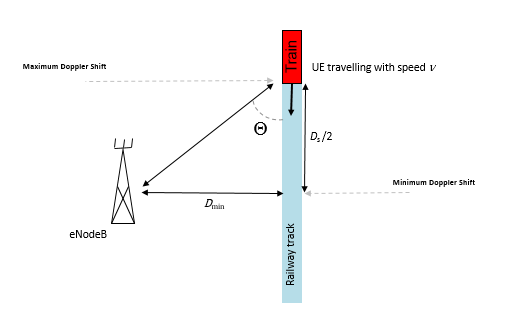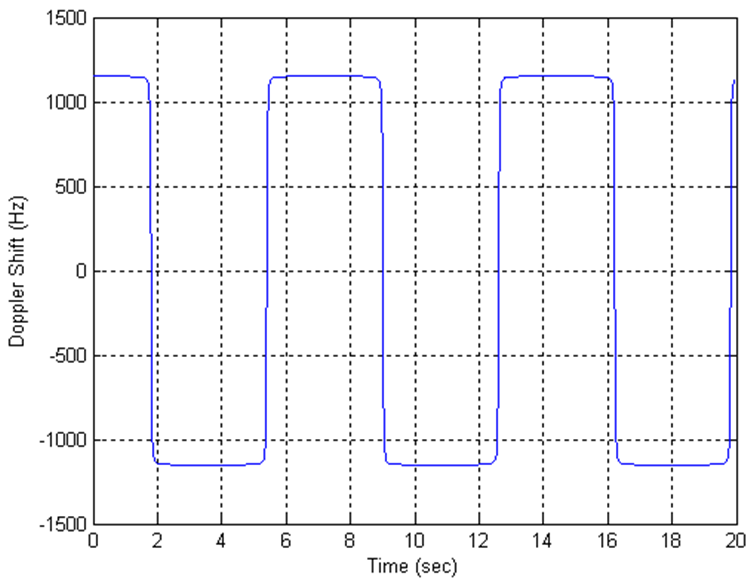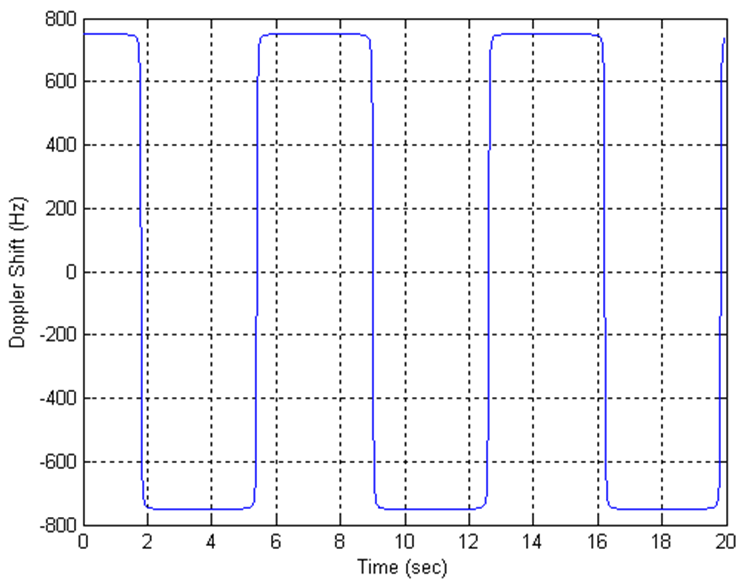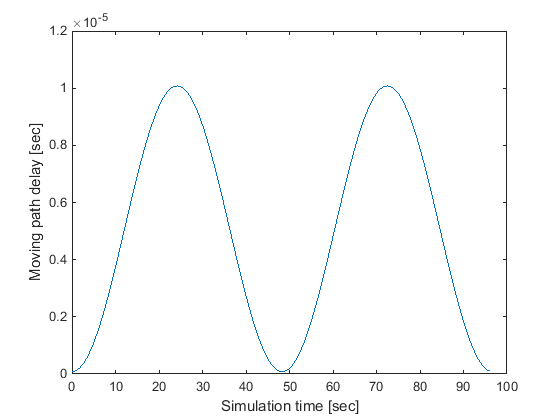Documentation

## Propagation Channel Models

The LTE Toolbox™ product provides a set of channel models for the test and verification of UE and eNodeB radio transmission and reception as defined in  and . The following channel models are available in the LTE Toolbox product.

• High speed train conditions

• Moving propagation conditions

The multipath fading channel model specifies the following three delay profiles.

• Extended Pedestrian A model (EPA)

• Extended Vehicular A model (EVA)

• Extended Typical Urban model (ETU)

These three delay profiles represent a low, medium, and high delay spread environment, respectively. The multipath delay profiles for these channels are shown in the following tables.

EPA Delay Profile

Excess tap delay (ns)Relative power (dB)
00.0
30–1.0
70–2.0
90–3.0
110–8.0
190–17.2
410–20.8

EVA Delay Profile

Excess tap delay (ns)Relative power (dB)
00.0
30–1.5
150–1.4
310–3.6
370–0.6
710–9.1
1090–7.0
1730–12.0
2510–16.9

ETU Delay Profile

Excess tap delay (ns)Relative power (dB)
0–1.0
50–1.0
120–1.0
2000.0
2300.0
5000.0
1600–3.0
2300–5.0
5000–7.0

All the taps in the preceding tables have a classical Doppler spectrum. In addition to multipath delay profile, a maximum Doppler frequency is specified for each multipath fading propagation condition, as shown in the following table.

Channel modelMaximum Doppler frequency
EPA 5Hz5 Hz
EVA 5Hz5 Hz
EVA 70Hz70 Hz
ETU 70Hz70 Hz
ETU 300Hz300 Hz

In the case of MIMO environments, a set of correlation matrices is introduced to model the correlation between UE and eNodeB antennas. These correlation matrices are introduced in MIMO Channel Correlation Matrices.

### High Speed Train Condition

The high speed train condition defines a non-fading propagation channel with single multipath component, the position of which is fixed in time. This single multipath represents the Doppler shift, which is caused due to a high speed train moving past a base station, as shown in the following figure.The expression ${D}_{s}/2$ is the initial distance of the train from eNodeB, and ${D}_{\mathrm{min}}$ is the minimum distance between eNodeB and the railway track. Both variables are measured in meters. The variable ν is the velocity of the train in meters per second. The Doppler shift due to a moving train is given in the following equation.

`${f}_{s}\left(t\right)={f}_{d}\mathrm{cos}\theta \left(t\right)$`

The variable ${f}_{s}\left(t\right)$ is the Doppler shift and ${f}_{d}$ is the maximum Doppler frequency. The cosine of angle $\theta \left(t\right)$ is given by the following equation.

`$\mathrm{cos}\theta \left(t\right)=\frac{{D}_{s}/2-vt}{\sqrt{{D}_{\mathrm{min}}{}^{2}+{\left({D}_{s}/2-vt\right)}^{2}}},0\le t\le {D}_{s}/v$`
`$\mathrm{cos}\theta \left(t\right)=\frac{-1.5{D}_{s}+vt}{\sqrt{{D}_{\mathrm{min}}{}^{2}+{\left(-1.5{D}_{s}+vt\right)}^{2}}},{D}_{s}/v`

For eNodeB testing, two high speed train scenarios are defined that use the parameters listed in the following table. The Doppler shift, ${f}_{s}\left(t\right)$, is calculated using the preceding equations and the parameters listed in the following table.

ParameterValue
Scenario 1Scenario 3
${D}_{s}$1,000 m300 m
${D}_{\mathrm{min}}$50 m2 m
ν350 km/hr300 km/kr
${f}_{d}$1,340 Hz1,150 Hz

Both of these scenarios result in Doppler shifts that apply to all frequency bands. The Doppler shift trajectory for scenario 1 is shown in the following figure.The Doppler shift trajectory for scenario 3 is shown in the following figure.For UE testing, the Doppler shift, ${f}_{s}\left(t\right)$, is calculated using the preceding equations and the parameters listed in the following table.

ParameterValue
${D}_{s}$300 m
${D}_{\mathrm{min}}$2 m
ν300 km/hr
${f}_{d}$750 Hz

These parameters result in the Doppler shift, applied to all frequency bands, shown in the following figure.### Moving Propagation Condition

The moving propagation channel in LTE defines a channel condition where the location of multipath components changes. The time difference between the reference time and the first tap, Δτ, is given by the following equation.

`$\Delta \tau =\frac{A}{2}\cdot \mathrm{sin}\left(\Delta \omega \cdot t\right)$`

The variable A represents the starting time in seconds and Δω represents angular rotation in radians per second.

### Note

Relative time between multipath components stays fixed.

The parameters for the moving propagation conditions are shown in the following table.

ParameterScenario 1Scenario 2
Channel modelETU200AWGN
UE speed120 km/hr350 km/hr
CP lengthNormalNormal
A10 μs10 μs
Δω0.04 s–10.13 s–1

Doppler shift only applies for generating fading samples for scenario 1. In scenario 2, a single non-fading multipath component with additive white gaussian noise (AWGN) is modeled. The location of this multipath component changes with time, according to the preceding equation.

An example of a moving channel with a single non-fading tap is shown in the following figure.### MIMO Channel Correlation Matrices

In MIMO systems, there is correlation between transmit and receive antennas. This depends on a number of factors such as the separation between antenna and the carrier frequency. For maximum capacity, it is desirable to minimize the correlation between transmit and receive antennas.

There are different ways to model antenna correlation. One technique makes use of correlation matrices to describe the correlation between multiple antennas both at the transmitter and the receiver. These matrices are computed independently at both the transmitter-receiver and then combined by means of a Kronecker product in order to generate a channel spatial correlation matrix.

Three different correlation levels are defined in .

1. low or no correlation

2. medium correlation

3. high correlation

The parameters α and β are defined for each level of correlation as shown in the following table of correlation values.

Low correlationMedium correlationHigh correlation
αβαβαβ
000.30.90.90.9

The independent correlation matrices at eNodeB and UE, ReNB and RUE, respectively, are shown for different set of antennas (1, 2 and 4) in the following table.

CorrelationOne antennaTwo antennasFour antennas
eNodeB

${R}_{eNB}=1$

${R}_{eNB}=\left(\begin{array}{cccc}1& {\alpha }^{1}{9}}& {\alpha }^{4}{9}}& \alpha \\ {\alpha }^{1}{9}}{}^{*}& 1& {\alpha }^{1}{9}}& {\alpha }^{4}{9}}\\ {\alpha }^{4}{9}}{}^{*}& {\alpha }^{1}{9}}{}^{*}& 1& {\alpha }^{1}{9}}\\ {\alpha }^{*}& {\alpha }^{4}{9}}{}^{*}& {\alpha }^{1}{9}}{}^{*}& 1\end{array}\right)$

UE

${R}_{UE}=1$

${R}_{UE}=\left(\begin{array}{cccc}1& {\beta }^{1}{9}}& {\beta }^{4}{9}}& \beta \\ {\beta }^{1}{9}}{}^{*}& 1& {\beta }^{1}{9}}& {\beta }^{4}{9}}\\ {\beta }^{4}{9}}{}^{*}& {\beta }^{1}{9}}{}^{*}& 1& {\beta }^{1}{9}}\\ {\beta }^{*}& {\beta }^{4}{9}}{}^{*}& {\beta }^{1}{9}}{}^{*}& 1\end{array}\right)$

The channel spatial correlation matrix, Rspat, is given by the following equation.

`${R}_{spat}={R}_{eNB}\otimes {R}_{UE}$`

The symbol ⊗ represents the Kronecker product. The values of the channel spatial correlation matrix, Rspat, for different matrix sizes are defined in the following table.

Matrix sizeRspat values
1×2 case

${R}_{spat}={R}_{UE}=\left(\begin{array}{cc}1& \beta \\ {\beta }^{*}& 1\end{array}\right)$

2×2 case

${R}_{spat}={R}_{eNB}\otimes {R}_{UE}=\left(\begin{array}{cc}1& \alpha \\ {\alpha }^{*}& 1\end{array}\right)\otimes \left(\begin{array}{cc}1& \beta \\ {\beta }^{*}& 1\end{array}\right)=\left(\begin{array}{cccc}1& \beta & \alpha & \alpha \beta \\ {\beta }^{*}& 1& \alpha {\beta }^{*}& \alpha \\ {\alpha }^{*}& {\alpha }^{*}\beta & 1& \beta \\ {\alpha }^{*}{\beta }^{*}& {\alpha }^{*}& {\beta }^{*}& 1\end{array}\right)$

4×2 case

${R}_{spat}={R}_{eNB}\otimes {R}_{UE}=\left(\begin{array}{cccc}1& {\alpha }^{1}{9}}& {\alpha }^{4}{9}}& \alpha \\ {\alpha }^{1}{9}}{}^{*}& 1& {\alpha }^{1}{9}}& {\alpha }^{4}{9}}\\ {\alpha }^{4}{9}}{}^{*}& {\alpha }^{1}{9}*}& 1& {\alpha }^{1}{9}}\\ {\alpha }^{*}& {\alpha }^{4}{9}}{}^{*}& {\alpha }^{1}{9}}{}^{*}& 1\end{array}\right)\otimes \left(\begin{array}{cc}1& \beta \\ {\beta }^{*}& 1\end{array}\right)$

4×4 case

${R}_{spat}={R}_{eNB}\otimes {R}_{UE}=\left(\begin{array}{cccc}1& {\alpha }^{1}{9}}& {\alpha }^{4}{9}}& \alpha \\ {\alpha }^{1}{9}}{}^{*}& 1& {\alpha }^{1}{9}}& {\alpha }^{4}{9}}\\ {\alpha }^{4}{9}}{}^{*}& {\alpha }^{1}{9}*}& 1& {\alpha }^{1}{9}}\\ {\alpha }^{*}& {\alpha }^{4}{9}}{}^{*}& {\alpha }^{1}{9}}{}^{*}& 1\end{array}\right)\otimes \left(\begin{array}{cccc}1& {\beta }^{1}{9}}& {\beta }^{4}{9}}& \beta \\ {\beta }^{1}{9}}{}^{*}& 1& {\beta }^{1}{9}}& {\beta }^{4}{9}}\\ {\beta }^{4}{9}}{}^{*}& {\beta }^{1}{9}*}& 1& {\beta }^{1}{9}}\\ {\beta }^{*}& {\beta }^{4}{9}}{}^{*}& {\beta }^{1}{9}}{}^{*}& 1\end{array}\right)$

 3GPP TS 36.101. “Evolved Universal Terrestrial Radio Access (E-UTRA); User Equipment (UE) Radio Transmission and Reception.” 3rd Generation Partnership Project; Technical Specification Group Radio Access Network. URL: https://www.3gpp.org.

 3GPP TS 36.104. “Evolved Universal Terrestrial Radio Access (E-UTRA); Base Station (BS) Radio Transmission and Reception.” 3rd Generation Partnership Project; Technical Specification Group Radio Access Network. URL: https://www.3gpp.org.